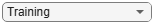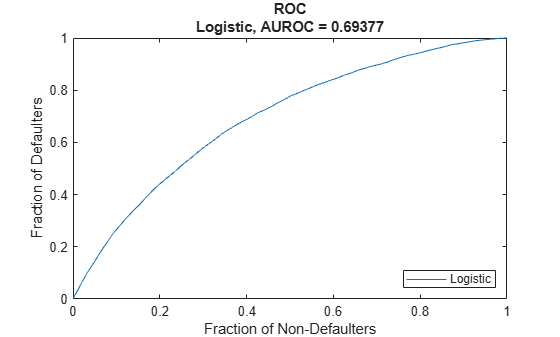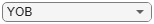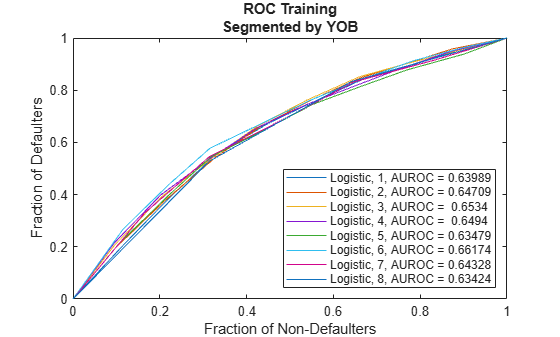modelDiscrimination

Compute AUROC and ROC data

Description

example

DiscMeasure = modelDiscrimination(pdModel,data) computes the area under the receiver operating characteristic curve (AUROC). modelDiscrimination supports segmentation and comparison against a reference model.

example

[DiscMeasure,DiscData] = modelDiscrimination(___,Name,Value) specifies options using one or more name-value pair arguments in addition to the input arguments in the previous syntax.

Examples

collapse all

This example shows how to use fitLifetimePDModel to fit data with a Logistic model and then generate the area under the receiver operating characteristic curve (AUROC) and ROC curve.

ID    ScoreGroup    YOB    Default    Year
__    __________    ___    _______    ____

1      Low Risk      1        0       1997
1      Low Risk      2        0       1998
1      Low Risk      3        0       1999
1      Low Risk      4        0       2000
1      Low Risk      5        0       2001
1      Low Risk      6        0       2002
1      Low Risk      7        0       2003
1      Low Risk      8        0       2004
Year     GDP     Market
____    _____    ______

1997     2.72      7.61
1998     3.57     26.24
1999     2.86      18.1
2000     2.43      3.19
2001     1.26    -10.51
2002    -0.59    -22.95
2003     0.63      2.78
2004     1.85      9.48

Join the two data components into a single data set.

data = join(data,dataMacro);
ID    ScoreGroup    YOB    Default    Year     GDP     Market
__    __________    ___    _______    ____    _____    ______

1      Low Risk      1        0       1997     2.72      7.61
1      Low Risk      2        0       1998     3.57     26.24
1      Low Risk      3        0       1999     2.86      18.1
1      Low Risk      4        0       2000     2.43      3.19
1      Low Risk      5        0       2001     1.26    -10.51
1      Low Risk      6        0       2002    -0.59    -22.95
1      Low Risk      7        0       2003     0.63      2.78
1      Low Risk      8        0       2004     1.85      9.48

Partition Data

Separate the data into training and test partitions.

nIDs = max(data.ID);
uniqueIDs = unique(data.ID);

rng('default'); % for reproducibility
c = cvpartition(nIDs,'HoldOut',0.4);

TrainIDInd = training(c);
TestIDInd = test(c);

TrainDataInd = ismember(data.ID,uniqueIDs(TrainIDInd));
TestDataInd = ismember(data.ID,uniqueIDs(TestIDInd));

Create a Logistic Lifetime PD Model

Use fitLifetimePDModel to create a Logistic model.

'AgeVar','YOB',...
'IDVar','ID',...
'LoanVars','ScoreGroup',...
'MacroVars',{'GDP','Market'},...
'ResponseVar','Default');
disp(pdModel)
Logistic with properties:

ModelID: "Logistic"
Description: ""
Model: [1x1 classreg.regr.CompactGeneralizedLinearModel]
IDVar: "ID"
AgeVar: "YOB"
LoanVars: "ScoreGroup"
MacroVars: ["GDP"    "Market"]
ResponseVar: "Default"

Display the underlying model.

disp(pdModel.Model)
Compact generalized linear regression model:
logit(Default) ~ 1 + ScoreGroup + YOB + GDP + Market
Distribution = Binomial

Estimated Coefficients:
Estimate        SE         tStat       pValue
__________    _________    _______    ___________

(Intercept)                  -2.7422      0.10136    -27.054     3.408e-161
ScoreGroup_Medium Risk      -0.68968     0.037286    -18.497     2.1894e-76
ScoreGroup_Low Risk          -1.2587     0.045451    -27.693    8.4736e-169
YOB                         -0.30894     0.013587    -22.738    1.8738e-114
GDP                         -0.11111     0.039673    -2.8006      0.0051008
Market                    -0.0083659    0.0028358    -2.9502      0.0031761

388097 observations, 388091 error degrees of freedom
Dispersion: 1
Chi^2-statistic vs. constant model: 1.85e+03, p-value = 0
disp(pdModel.Model.Coefficients)
Estimate        SE         tStat       pValue
__________    _________    _______    ___________

(Intercept)                  -2.7422      0.10136    -27.054     3.408e-161
ScoreGroup_Medium Risk      -0.68968     0.037286    -18.497     2.1894e-76
ScoreGroup_Low Risk          -1.2587     0.045451    -27.693    8.4736e-169
YOB                         -0.30894     0.013587    -22.738    1.8738e-114
GDP                         -0.11111     0.039673    -2.8006      0.0051008
Market                    -0.0083659    0.0028358    -2.9502      0.0031761

Model Discrimination to Generate AUROC and ROC

Model "discrimination" measures how effectively a model ranks customers by risk. You can use the AUROC and ROC outputs to determine whether customers with higher predicted PDs actually have higher risk in the observed data.

DataSetChoice ="Training";
if DataSetChoice=="Training"
Ind = TrainDataInd;
else
Ind = TestDataInd;
end

DiscMeasure = modelDiscrimination(pdModel,data(TrainDataInd,:),'DataID',DataSetChoice);
disp(DiscMeasure)
AUROC
_______

Logistic, Training    0.69377

Visualize the ROC for the Logistic model using modelDiscriminationPlot.

modelDiscriminationPlot(pdModel,data(TrainDataInd,:));Data can be segmented to get the AUROC per segment and the corresponding ROC data.

SegmentVar ="YOB";
DiscMeasure = modelDiscrimination(pdModel,data(Ind,:),'SegmentBy',SegmentVar,'DataID',DataSetChoice);
disp(DiscMeasure)
AUROC
_______

Logistic, YOB=1, Training    0.63989
Logistic, YOB=2, Training    0.64709
Logistic, YOB=3, Training     0.6534
Logistic, YOB=4, Training     0.6494
Logistic, YOB=5, Training    0.63479
Logistic, YOB=6, Training    0.66174
Logistic, YOB=7, Training    0.64328
Logistic, YOB=8, Training    0.63424

Visualize the ROC segmented by YOB, ScoreGroup, or Year using modelDiscriminationPlot.

modelDiscriminationPlot(pdModel,data(Ind,:),'SegmentBy',SegmentVar,'DataID',DataSetChoice);Input Arguments

collapse all

Probability of default model, specified as a Logistic, Probit, or Cox object previously created using fitLifetimePDModel.

Note

The 'ModelID' property of the pdModel object is used as the identifier or tag for pdModel.

Data Types: object

Data, specified as a NumRows-by-NumCols table with projected predictor values to make lifetime predictions. The predictor names and data types must be consistent with the underlying model.

Data Types: table

Name-Value Arguments

Specify optional comma-separated pairs of Name,Value arguments. Name is the argument name and Value is the corresponding value. Name must appear inside quotes. You can specify several name and value pair arguments in any order as Name1,Value1,...,NameN,ValueN.

Example: [PerfMeasure,PerfData] = modelDiscrimination(pdModel,data(Ind,:),'DataID',"DataSetChoice")

Data set identifier, specified as the comma-separated pair consisting of 'DataID' and a character vector or string.

Data Types: char | string

Name of a column in the data input, not necessarily a model variable, to be used to segment the data set, specified as the comma-separated pair consisting of 'SegmentBy' and a character vector or string.

One AUROC value is reported for each segment and the corresponding ROC data for each segment is returned in the PerfData optional output.

Data Types: char | string

Conditional PD values predicted for data by the reference model, specified as the comma-separated pair consisting of 'ReferencePD' and a NumRows-by-1 numeric vector. The modelDiscrimination output information is reported for both the pdModel object and the reference model.

Data Types: double

Identifier for the reference model, specified as the comma-separated pair consisting of 'ReferenceID' and a character vector or string. 'ReferenceID' is used in the modelDiscrimination output for reporting purposes.

Data Types: char | string

Output Arguments

collapse all

AUROC information for each model and each segment., returned as a table. DiscMeasure has a single column named 'AUROC' and the number of rows depends on the number of segments and whether you use a ReferenceID for a reference model and ReferencePD for reference data. The row names of DiscMeasure report the model IDs, segment, and data ID.

ROC data for each model and each segment, returned as a table. There are three columns for the ROC data, with column names 'X', 'Y', and 'T', where the first two are the X and Y coordinates of the ROC curve, and T contains the corresponding thresholds.

If you use SegmentBy, the function stacks the ROC data for all segments and DiscData has a column with the segmentation values to indicate where each segment starts and ends.

If reference model data is given using ReferenceID and ReferencePD, the DiscData outputs for the main and reference models are stacked, with an extra column 'ModelID' indicating where each model starts and ends.

collapse all

Model Discrimination

Model discrimination measures the risk ranking.

Higher-risk loans should get higher predicted probability of default (PD) than lower-risk loans. The modelDiscrimination function computes the Area Under the Receiver Operator Characteristic curve (AUROC), sometimes called simply the Area Under the Curve (AUC). This metric is between 0 and 1 and higher values indicate better discrimination.